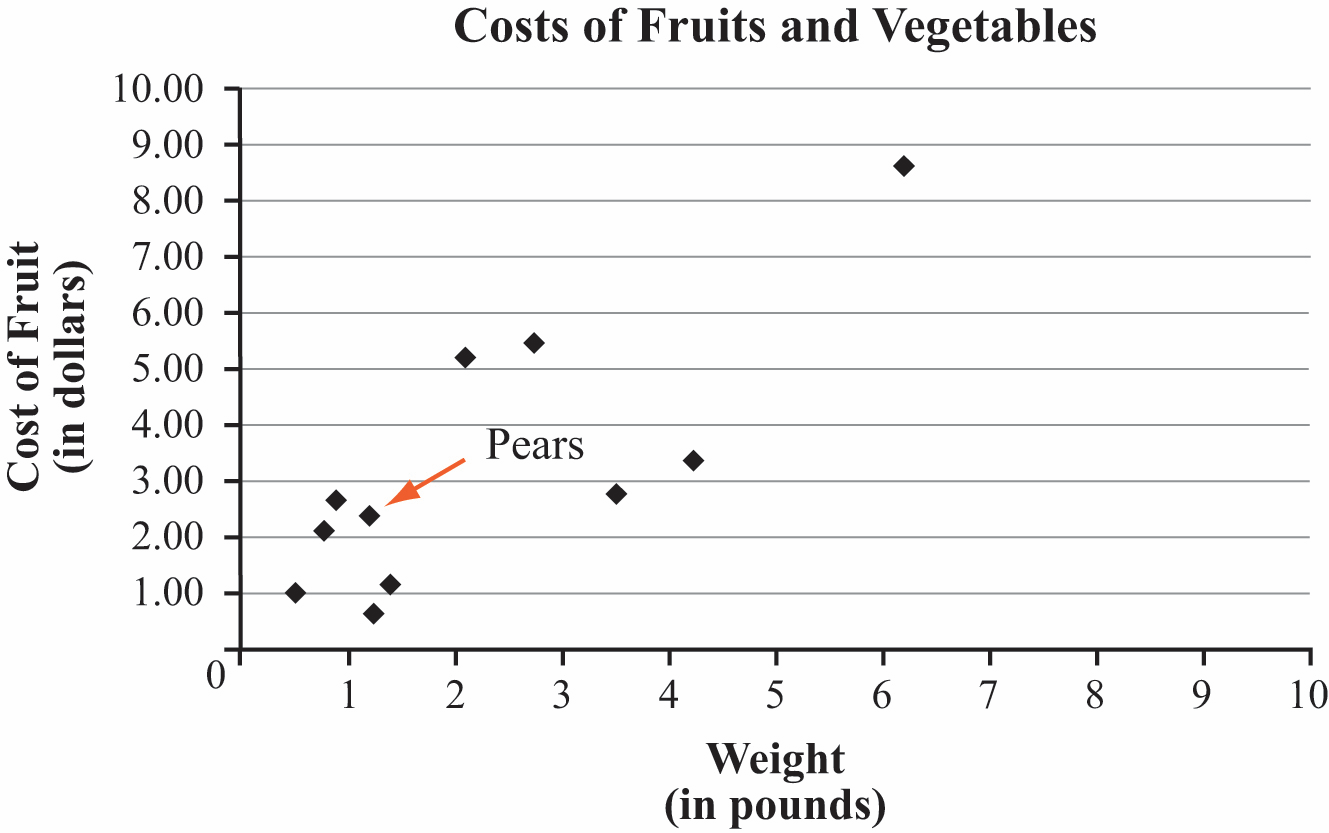# Proportional Relationships

## Objective

Use proportional reasoning to solve real-world, multi-step problems.

## Common Core Standards

### Core Standards

?

• 7.RP.A.1 — Compute unit rates associated with ratios of fractions, including ratios of lengths, areas and other quantities measured in like or different units. For example, if a person walks 1/2 mile in each 1/4 hour, compute the unit rate as the complex fraction 1/2/1/4 miles per hour, equivalently 2 miles per hour.

• 7.RP.A.2 — Recognize and represent proportional relationships between quantities.

• 7.RP.A.3 — Use proportional relationships to solve multistep ratio and percent problems. Examples: simple interest, tax, markups and markdowns, gratuities and commissions, fees, percent increase and decrease, percent error.

?

• 6.RP.A.3

## Criteria for Success

?

1. Solve multi-step real-world problems involving rates and ratios using proportional reasoning and a variety of strategies (MP.4).
2. Organize information and map out a solution process for a multi-step problem (MP.1).
3. Decontextualize situations to represent them in various forms such as equations, graphs, tables, etc. and re-contextualize representations to explain their meanings as they relate to the situation (MP.2).

## Tips for Teachers

?

• This is a flex lesson that can be used in a variety of ways, depending on the individual class. There are no anchor problems, as teachers can determine what specific concepts are best to look at with the whole class.
• The Problem Set Guidance is a collection of activities and problems utilizing skills from the entire unit that may be used for this day, including a Desmos activity, three-act tasks, and other modeling tasks.

## Problem Set

?The following resources include problems and activities aligned to the objective of the lesson that can be used to create your own problem set.

?

The graph shows the weights and costs of the fruits and vegetables Tyrus bought at the store. For example, Tyrus bought 1.2 pounds of pears for \$2.39.1. Circle all points with a higher cost per pound than the pears. Explain why you circled those points.
2. Put an “x” on three points with the same cost per pound. Explain how you know.
3. Next week, pears will cost two times as much per pound and Tyrus will buy the same amount. What will happen to the location of the point on the graph representing pears? Explain how you know.

#### References

Achieve the Core Grade 7 Proportional Relationships Mini-Assessment (2016 version)

Grade 7 Proportional Relationships Mini-Assessment (2016 version) by Student Achievement Partners is made available by Achieve the Core under the CC0 1.0 license. Accessed Aug. 2, 2017, 1:37 p.m..

?